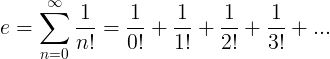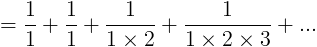# Euler’s Constant

## Related Post

e constant or Euler’s number is a mathematical constant. The e constant is real and irrational number.

e = 2.718281828459…

The e constant is defined as the limit:The e constant is defined as the limit:The e constant is defined as the infinite series:Properties of e

Reciprocal of e

The reciprocal of e is the limit:Derivatives of e

The derivative of the exponential function is the exponential function:

(e x)’ = ex

The derivative of the natural logarithm function is the reciprocal function:

(loge x)‘ = (ln x)’ = 1/x

Integrals of e

The indefinite integral of the exponential function ex is the exponential function ex.

ex dx = ex+c

The indefinite integral of the natural logarithm function loge x is:

∫ loge x dx = ∫ lnx dx = x ln x – x +c

The definite integral from 1 to e of the reciprocal function 1/x is 1:Base e logarithm

The natural logarithm of a number x is defined as the base e logarithm of x:

ln x = loge x

Exponential function

The exponential function is defined as:

f (x) = exp(x) = ex

Euler’s formula

The complex number e has the identity:

e = cos(θ) + i sin(θ)

i is the imaginary unit (the square root of -1). θ is any real number.

The e constant can be approximately calculated by using the infinite series:= 1 + 1 + 0.5 + 0.166667 + …

= 2.71828182…

≈ 2.71828183

2.71828183 is an approximated value of the e constant. The e constant has an infinite number of digits.